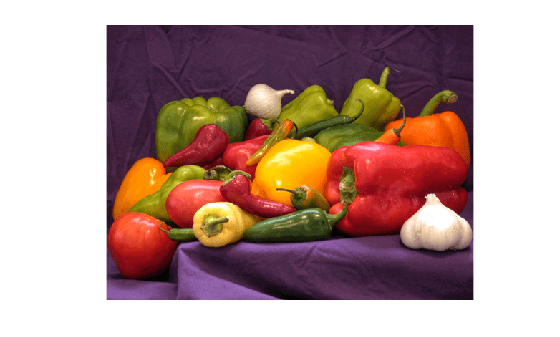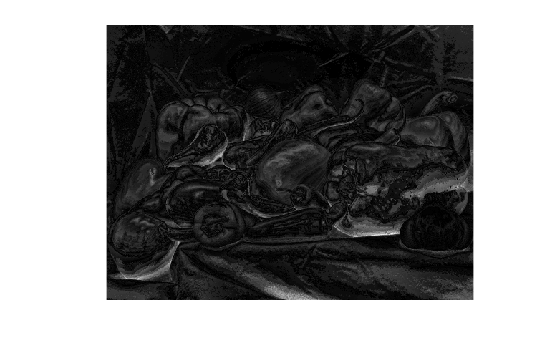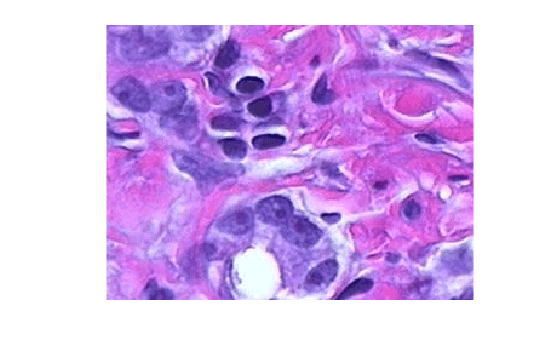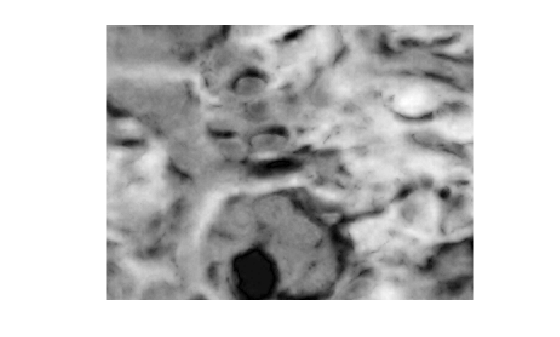deltaE

Color difference based on CIE76 standard

Description

example

dE = deltaE(I1,I2) calculates the color difference between two RGB images or sets of colors using the CIE76 standard.

example

dE = deltaE(I1,I2,'isInputLab',isLab) also specifies whether the input color data is in the RGB color space or the L*a*b* color space.

Examples

collapse all

Specify two RGB color values.

pureRed = uint8([255 0 0]);
darkRed = uint8([255 10 50]);

Calculate the color difference of the colors.

dE = deltaE(pureRed,darkRed)
dE = single
18.6206

Read a color image into the workspace.

imshow(I1)Alter the local color contrast in the image.

I2 = localcontrast(I1);
imshow(I2)Calculate the color difference of the images.

dE = deltaE(I1,I2);

Display the color difference as an image. The maximum value of dE exceeds the range [0, 1] expected of images of data type single, so display the image using the full display range of the data. Bright pixels indicate a large color difference and therefore a larger amount of contrast enhancement.

imshow(dE,[])Read and display an image of tissue stained with hemotoxylin and eosin (H&E).

imshow(he)Convert the image to the L*a*b* color space.

lab = rgb2lab(he);

Make a copy of the image, then increase the signal of the a* channel. Red tones in the image become more saturated while the image overall brightness and the blue tones are unchanged.

lab2 = lab;
scaleFactor = 1.2;
lab2(:,:,2) = scaleFactor*lab(:,:,2);

Calculate the color difference of the original and enhanced image in the L*a*b* color space.

dE = imcolordiff(lab,lab2,'isInputLab',true);

Display the color difference as an image. Scale the display range to match the range of pixel values in dE. Bright regions indicate the greatest color difference and correspond with the pink regions of tissue.

imshow(dE,[])Input Arguments

collapse all

First set of color data, specified as an m-by-n-by-3 numeric array representing an image or a c-by-3 numeric matrix representing a set of c colors. I1 and I2 must be the same size with values in the same color space.

By default, the deltaE function interprets the color data as RGB color values. To calculate the color difference in the L*a*b* color space, specify the isLab argument as true. L*a*b* color values can be of data type single or double only.

Data Types: single | double | uint8 | uint16

Second set of color data, specified as an m-by-n-by-3 numeric array representing an image or a c-by-3 numeric matrix representing a set of c colors. I1 and I2 must be the same size with values in the same color space.

By default, the deltaE function interprets the color data as RGB color values. To calculate the color difference in the L*a*b* color space, specify the isLab argument as true. L*a*b* color values can be of data type single or double only.

Data Types: single | double | uint8 | uint16

Color values are in the L*a*b* color space, specified as a numeric or logical 0 (false) or 1 (true).

Output Arguments

collapse all

Color difference (delta E), returned as one of the following.

• An m-by-n matrix when the input color data I1 and I2 represent images

• A c-element column vector when I1 and I2 represent a set of c colors

If I1 or I2 is of data type double, then dE is of data type double. Otherwise, dE is of data type single.

Data Types: single | double

Tips

• To improve the accuracy of the color difference calculation, use the imcolordiff function. This function follows the CIE94 and CIEDE2000 standards and offers parameters to improve perceptual uniformity for different applications.